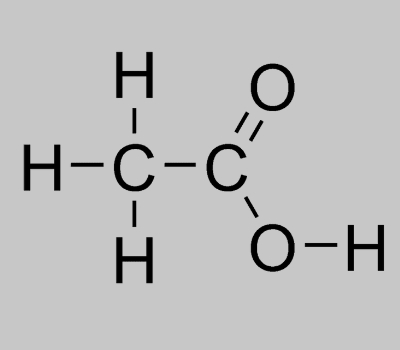# Vinegar Formula

When we talk about Vinegar formula there are many questions that arise sometimes. Since vinegar isn’t a single chemical substance and includes a mixture of several different substances in the form of a solution there are chances of presenting each with their own formula. However, this is not done as it can be ambiguous. The structural formula for acetic acid (the chemical name of vinegar) is usually taken.

## Vinegar Chemical Formula

Since acetic acid is the primary component present, the chemical formula of vinegar is the same. Notably, the molecular formula of acetic acid is considered because it tells us about the actual structure of the molecules. It further denotes the presence of a carboxylic group which is an important aspect in certain cases while dealing with substances. The formula is basically represented as CH3COOH.

 Formula CH3COOH / C2H4O2 Molar Mass 60.052 g/mol Density approximately 0.96 g/mL Melting Point 16.6 °C Boiling Point 118.1 °C

### Vinegar Structural Formula

The structural formula of vinegar can be pictured by understanding the chemical formula of acetic acid. We can see that the first carbon atom is connected to three hydrogen atoms while the second carbon atom is bonded together with an oxygen atom and a hydroxyl group. A representation of vinegar structural formula is given below.Keep visiting BYJU’S to access different formulas of important chemical compounds.